Processing ......FreeComputerBooks.com Links to Free Computer, Mathematics, Technical Books all over the World

Computer Age Statistical Inference: Algorithms, Evidence, and Data Science
Top Free Computer Networking Books 🌠 - 100% Free or Open Source!
• Title: Computer Age Statistical Inference: Algorithms, Evidence, and Data Science
• Author(s) Bradley Efron and Trevor Hastie
• Publisher: Cambridge University Press; 1 edition (July 21, 2016); eBook (Stanford University 2016)
• Hardcover: 495 pages
• eBook: PDF (493 pages)
• Language: English
• ISBN-10: 1107149894
• ISBN-13: 978-1107149892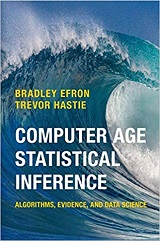Book Description

A masterful guide to how the inferential bases of classical statistics can provide a principled disciplinary frame for the data science of the twenty-first century. Every aspiring data scientist should carefully study this book, use it as a reference, and carry it with them everywhere.

This book takes us on an exhilarating journey through the revolution in data analysis following the introduction of electronic computation in the 1950s. Beginning with classical inferential theories - Bayesian, frequentist, Fisherian - individual chapters take up a series of influential topics: survival analysis, logistic regression, empirical Bayes, the jackknife and bootstrap, random forests, neural networks, Markov chain Monte Carlo, inference after model selection, and dozens more.

The distinctly modern approach integrates methodology and algorithms with statistical inference. The book ends with speculation on the future direction of statistics and data science.

• Bradley Efron is Max H. Stein Professor, Professor of Statistics, and Professor of Biomedical Data Science at Stanford University, California.
• Trevor Hastie is John A. Overdeck Professor, Professor of Statistics, and Professor of Biomedical Data Science at Stanford University, California. He is coauthor of Elements of Statistical Learning, a key text in the field of modern data analysis.
Reviews, Ratings, and Recommendations: Related Book Categories: Read and Download Links:Similar Books:
•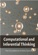Computational and Inferential: The Foundations of Data Science

Step by step, you'll learn how to leverage algorithmic thinking and the power of code, gain intuition about the power and limitations of current machine learning methods, and effectively apply them to real business problems.

•Statistical Inference for Everyone (Brian S Blais)

Approaching an introductory statistical inference textbook in a novel way, this book walks through a simple introduction to probability, and then applies those principles to all problems of inference.

•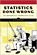Statistics Done Wrong: The Woefully Complete Guide (Reinhart)

Scientific progress depends on good research, and good research needs good statistics. But statistical analysis is tricky to get right, even for the best and brightest of us. You'd be surprised how many scientists are doing it wrong.

•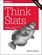Think Stats, 2nd Edition: Exploratory Data Analysis in Python

This concise introduction shows you how to perform statistical analysis computationally, rather than mathematically, with programs written in Python. You'll become familiar with distributions, the rules of probability, visualization, and many other tools and concepts.

•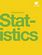Introductory Statistics (OpenStax)

This book is geared toward students majoring in fields other than math or engineering. This text assumes students have been exposed to intermediate algebra, and it focuses on the applications of statistical knowledge rather than the theory behind it.

•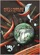Mostly Harmless Statistics (Rachel L. Webb)

This text is for an introductory level probability and statistics course with an intermediate algebra prerequisite. The focus of the text follows the American Statistical Association's Guidelines for Assessment and Instruction in Statistics Education (GAISE).

•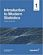Introduction to Modern Statistics (Mine Çetinkaya-Rundel, et al.)

This book puts a heavy emphasis on exploratory data analysis and provides a thorough discussion of simulation-based inference using randomization and bootstrapping, followed by a presentation of the related Central Limit Theorem based approaches.

•Foundations in Statistical Reasoning (Pete Kaslik)

This book is designed for students taking an introductory statistics class. The emphasis throughout the entire book is on how to make decisions with only partial evidence. It focuses on the thought process.

•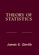Theory of Statistics (James E. Gentle)

This book is directed toward students for whom mathematical statistics is or will become an important part of their lives. It covers classical likelihood, Bayesian, and permutation inference; an introduction to basic asymptotic distribution theory; and modern topics.

•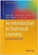Introduction to Statistical Learning: with Applications in Python

This book covers the same materials as Introduction to Statistical Learning: with Applications in R (ISLR) but with labs implemented in Python. These labs will be useful both for Python novices, as well as experienced users.

•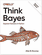O'Reilly® Think Bayes: Bayesian Statistics in Python

If you know how to program, you're ready to tackle Bayesian statistics. With this book, you'll learn how to solve statistical problems with Python code instead of mathematical formulas, using discrete probability distributions rather than continuous mathematics.

•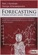Forecasting: Principles and Practice (Rob J. Hyndman, et al.)

This textbook provides a comprehensive introduction to forecasting methods and presents enough information about each method for readers to use them sensibly. Examples use R with many data sets taken from the authors' own consulting experience.

•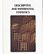Foundations of Descriptive and Inferential Statistics (H. van Elst)

This book aim to provide an accessible though technically solid introduction to the logic of systematical analyses of statistical data to both undergraduate and postgraduate students, in particular in the Social Sciences, Economics, and the Financial Services.

•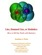Lies, Damned Lies: How to Tell the Truth with Statistics

The goal is to help you learn How to Tell the Truth with Statistics and, therefore, how to tell when others are telling the truth ... or are faking their "news". Covers Data Analysis, Binomial and normal models, Sample statistics, confidence intervals, hypothesis tests, etc.

•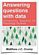Answering Questions with Data : Introductory Statistics

This is a free textbook teaching introductory statistics for undergraduates. Students will learn to select an appropriate data analysis technique, carry out the analysis, and draw appropriate conclusions.

•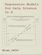Regression Models for Data Science in R (Brian Caffo)

The book gives a rigorous treatment of the elementary concepts of regression models from a practical perspective. The ideal reader for this book will be quantitatively literate and has a basic understanding of statistical concepts and R programming.

•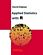Applied Statistics with R (David Dalpiaz)

This book provides an integrated treatment of statistical inference techniques in data science using the R Statistical Software. It provides a much-needed, easy-to-follow introduction to statistics and the R programming language.

•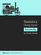Statistics Using Excel Succinctly (Charles Zaiontz)

This book illustrates the capabilities of Microsoft Excel to teach applied statistics effectively. It is a step-by-step exercise-driven guide for students and practitioners who need to master Excel to solve practical statistical problems

Book Categories
 :All CategoriesTop Free BooksRecent BooksMiscellaneous BooksComputer EngineeringComputer LanguagesComputer ScienceData Science/DatabasesJava and Java EE (J2EE)Linux and UnixMathematicsMicrosoft and .NETMobile ComputingNetworking and CommunicationsSoftware EngineeringSpecial TopicsWeb Programming
Other Categories